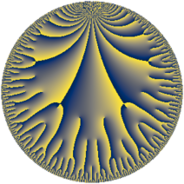# Properties

 Label 12.22.bLevel $12$ Weight $22$ Character orbit 12.b Rep. character $\chi_{12}(11,\cdot)$ Character field $\Q$ Dimension $40$ Newform subspaces $1$ Sturm bound $44$ Trace bound $0$

# Related objects

## Defining parameters

 Level: $$N$$ $$=$$ $$12 = 2^{2} \cdot 3$$ Weight: $$k$$ $$=$$ $$22$$ Character orbit: $$[\chi]$$ $$=$$ 12.b (of order $$2$$ and degree $$1$$) Character conductor: $$\operatorname{cond}(\chi)$$ $$=$$ $$12$$ Character field: $$\Q$$ Newform subspaces: $$1$$ Sturm bound: $$44$$ Trace bound: $$0$$

## Dimensions

The following table gives the dimensions of various subspaces of $$M_{22}(12, [\chi])$$.

Total New Old
Modular forms 44 44 0
Cusp forms 40 40 0
Eisenstein series 4 4 0

## Trace form

 $$40 q + 1212040 q^{4} - 73477608 q^{6} + 4862757768 q^{9} + O(q^{10})$$ $$40 q + 1212040 q^{4} - 73477608 q^{6} + 4862757768 q^{9} + 70993722608 q^{10} + 230311800264 q^{12} + 532697931824 q^{13} - 3296861950688 q^{16} - 38138435545488 q^{18} + 6345498814320 q^{21} + 345245332418448 q^{22} + 162733136355360 q^{24} - 2870634573953208 q^{25} + 3010485857135664 q^{28} + 5225832293639088 q^{30} - 7282205643351936 q^{33} - 11653770049992256 q^{34} - 18580578856893912 q^{36} - 42230886115147792 q^{37} - 89660451263290816 q^{40} - 196777060056297264 q^{42} + 490656937607621376 q^{45} - 24590270471085024 q^{46} - 1070121602451491040 q^{48} - 1521600851011396808 q^{49} + 1474695233111072240 q^{52} - 107978656785090264 q^{54} - 410005705945522032 q^{57} + 9260173307858336144 q^{58} + 6491621502020133696 q^{60} - 5700218795673195856 q^{61} - 2949437838551773568 q^{64} + 1836224451373338192 q^{66} + 25444406037298107648 q^{69} - 24886444039106795232 q^{70} - 27969226308253542336 q^{72} + 101891967257574779024 q^{73} + 22386121373924673552 q^{76} + 76385487692811283152 q^{78} - 476218176140556806040 q^{81} + 1864686882869863520 q^{82} + 311685180307346738352 q^{84} - 605170615993311678464 q^{85} + 15063645347500158144 q^{88} - 159737520743388593232 q^{90} + 682248293569820157360 q^{93} + 242051617770611035200 q^{94} - 1187951481218430818688 q^{96} + 196191301644672463568 q^{97} + O(q^{100})$$

## Decomposition of $$S_{22}^{\mathrm{new}}(12, [\chi])$$ into newform subspaces

Label Dim $A$ Field CM Traces $q$-expansion
$a_{2}$ $a_{3}$ $a_{5}$ $a_{7}$
12.22.b.a $40$ $33.537$ None $$0$$ $$0$$ $$0$$ $$0$$